Win up to 100% scholarship on Aakash BYJU'S JEE/NEET courses with ABNAT Win up to 100% scholarship on Aakash BYJU'S JEE/NEET courses with ABNAT

# Limits, Continuity and Differentiability - Evaluations and Examples

The limit concept is certainly indispensable for the development of analysis, for convergence and divergence of infinite series also depend on this concept. The theory of limits and then defining continuity, differentiability and the definite integral in terms of the limit concept is successfully executed by mathematicians. In this section, you will study this concept in detail with the help of solved examples.

## What Are Limits?

The expression

$$\begin{array}{l}\underset{x\to c}{\mathop{\lim }}\,\,f(x)=L\end{array}$$
means that f(x) can be as close to L as desired by making x sufficiently close to ‘C’. In such a case, we say that the limit of f, as x approaches to C, is L.

The neighbourhood of a point:

Let ‘a’ be real number and ‘h; is very close to ‘O’, then

The left-hand limit will be obtained when x = a – h or x -> a

Similarly, the right-hand limit will be obtained when x = a + h or x ->  a+

Related Concepts:

### Existence of Limit

The limit will exist if the following conditions get fulfilled:

(a)

$$\begin{array}{l}\underset{x\to {{a}^{-}}}{\mathop{\lim }} = f(x) =\underset{x\to {{a}^{+}}}{\mathop{\lim }} \end{array}$$
, i.e., LHL = RHL

(b) Both LHL & RHL should be finite.

Examples

• $$\begin{array}{l}\underset{x\to 1}{\mathop{\lim }}\,\,{{x}^{2}}+1={{1}^{2}}+1=2\end{array}$$
• $$\begin{array}{l}\underset{x\to 0}{\mathop{\lim }}\,\,{{x}^{2}}-x={{0}^{2}}-0=0\end{array}$$
• $$\begin{array}{l}\underset{x\to 2}{\mathop{\lim }}\,\,\frac{{{x}^{2}}-4}{x+3}=\frac{4-4}{2+3}=0\end{array}$$

(c) In limits, we have in determinant forms such as

$$\begin{array}{l}\frac{0}{0},\frac{\infty }{\infty },0\times \infty,\infty \times \infty,{{1}^{\infty }},0{}^\circ,\infty {}^\circ\end{array}$$

In these cases, we try to simplify the problem into a valid function.

### Watch This Video for More Reference

#### Calculus Problems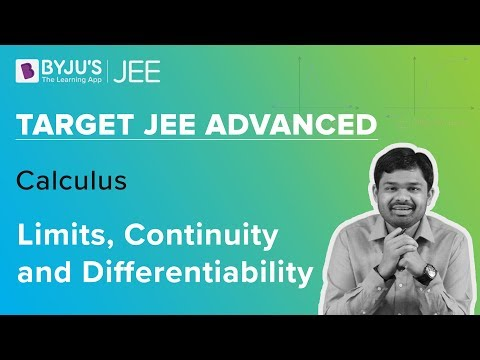#### Expected Questions and Solutions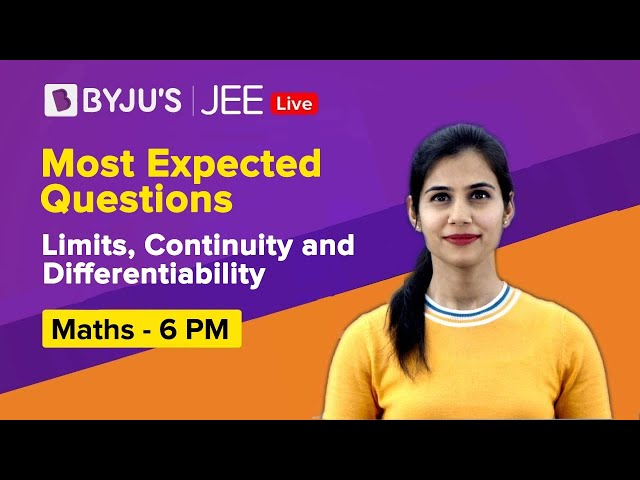### Use of Expansion in Evaluating Limits

Some Important Expansions

1. $$\begin{array}{l} \log (1+x)=x-\frac{{{x}^{2}}}{2}+\frac{{{x}^{3}}}{3}-\frac{{{x}^{4}}}{4}+…….\end{array}$$
2. $$\begin{array}{l}{{e}^{x}}=1+x+\frac{{{x}^{2}}}{2!}+\frac{{{x}^{3}}}{3!}-\frac{{{x}^{4}}}{4!}+…….\end{array}$$
3. $$\begin{array}{l}{{a}^{x}}=1+x\log a+\frac{{{x}^{2}}}{2!}{(log\;a)}^{2}+…….\end{array}$$
4. $$\begin{array}{l}\sin x=x-\frac{{{x}^{3}}}{3!}+\frac{{{x}^{5}}}{5!}……\end{array}$$
5. $$\begin{array}{l}\cos x=1-\frac{{{x}^{2}}}{2!}+\frac{{{x}^{4}}}{4!}……\end{array}$$
6. $$\begin{array}{l}\tan x=x+\frac{{{x}^{3}}}{3}+\frac{2}{15}{{x}^{5}}+……\end{array}$$

### Some Important limits

1. $$\begin{array}{l}\underset{x\to 0}{\mathop{\lim }}\,\,\,\,\frac{\sin x}{x}=1\end{array}$$
2. $$\begin{array}{l}\underset{x\to 0}{\mathop{\lim }}\,\,\,\,\frac{1-\cos x}{{{x}^{2}}}=\frac{1}{2}\end{array}$$
3. $$\begin{array}{l}\underset{x\to 0}{\mathop{\lim }}\,\,\,\,\frac{\tan x}{x}=1\end{array}$$
4. $$\begin{array}{l}\underset{x\to 0}{\mathop{\lim }}\,\,\,\,\frac{{{e}^{x}}-1}{x}=1\end{array}$$
5. $$\begin{array}{l}\underset{x\to 0}{\mathop{\lim }}\,\,\,\,\frac{\log (1+x)}{x}=1\end{array}$$

Example: Solve

$$\begin{array}{l}\underset{x\to 0}{\mathop{\lim }}\,\,\,\,\frac{\sin x-x}{{{x}^{3}}}\end{array}$$

Solution: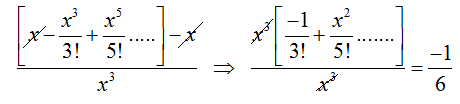### Evaluation of Algebraic Limits

#### Direct substitution method

Example 1:

$$\begin{array}{l}\underset{x\to 1}{\mathop{\lim }}\,\,(3{{x}^{2}}+4x+5)\end{array}$$
= 3(1)2 + 4(1) + 5 = 12

Example 2:

$$\begin{array}{l}\underset{x\to 2}{\mathop{\lim }}\,\,\frac{{{x}^{2}}-4}{x+3}=\frac{4-4}{2+3}=\frac{0}{5}=0\end{array}$$

#### Factorisation method

Example:

$$\begin{array}{l}\underset{x\to 2}{\mathop{\lim }}\,\,\frac{{{x}^{2}}-5x+6}{{{x}^{2}}-4}\end{array}$$

Solution:

$$\begin{array}{l}\underset{x\to 2}{\mathop{\lim }}\,\,\frac{(x-2)(x-3)}{(x+2)(x-2)}=\underset{x\to 2}{\mathop{\lim }}\,\frac{x-3}{x+2}\end{array}$$
$$\begin{array}{l}=\frac{-1}{4}\end{array}$$

#### Rationalisation method

Example:

$$\begin{array}{l}\underset{x\to 0}{\mathop{\lim }}\,\,\frac{\sqrt{2+x}-\sqrt{2}}{x}\end{array}$$

Solution:

$$\begin{array}{l}\underset{x\to 0}{\mathop{\lim }}\,\,\frac{\left( \sqrt{2+x}-\sqrt{2} \right)\left( \sqrt{2+x}+\sqrt{2} \right)}{x\left( \sqrt{2+x}+\sqrt{2} \right)}\end{array}$$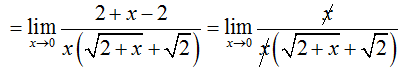$$\begin{array}{l}=\frac{1}{2\sqrt{2}}\end{array}$$

Using Result:

$$\begin{array}{l}\underset{x\to a}{\mathop{\lim }}\,\frac{{{x}^{n}}-{{a}^{n}}}{x-a}=n{{a}^{n-1}}\end{array}$$

Example:

$$\begin{array}{l}\underset{x\to 2}{\mathop{\lim }}\,\frac{{{x}^{10}}-{{2}^{10}}}{{{x}^{5}}-{{2}^{5}}}\end{array}$$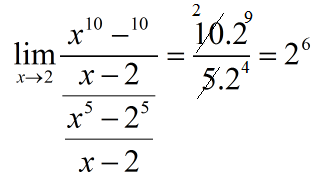## Continuity

### What Is Continuity?

A continuous function is a function for which small changes in the input results in small changes in the output. Otherwise, a function is said to be discontinuous.

A function f(x) is said to be continuous at x = a if

$$\begin{array}{l}\underset{x\to a}{\mathop{\lim }}\,f(x)=\underset{x\to {{a}^{+}}}{\mathop{\lim }}\,f(x)=f(a)\end{array}$$

i.e., LHL = RHL = value of the function at x = a

Else, a function f(x) is said to be a discontinuous function.

Example 1:

$$\begin{array}{l}f(x) = \frac{1}{2\sin x-1},\end{array}$$
discuss the continuity or discontinuity.

Solution: Clearly, the function will be not defined for

$$\begin{array}{l}\sin x=\frac{1}{2}=\sin \frac{\pi }{6}\end{array}$$

Function is discontinuous for

$$\begin{array}{l}x=n\,\pi +{{(-1)}^{n}}\frac{\pi }{6}\end{array}$$
.

Example 2: What value must be assigned to K so that the function

$$\begin{array}{l}f(x)=\left\{\begin{matrix} \frac{x^4-256}{x-4} & , & x\neq 4\\ k & , & x=4 \end{matrix}\right.\end{array}$$
is continuous at x = 4?

Solution:

$$\begin{array}{l}f(4)=\underset{x\to 4}{\mathop{\lim }}\,\,\,\,\,\frac{{{x}^{4}}-256}{x-4}=\underset{x\to 0}{\mathop{\lim }}\,\frac{{{x}^{4}}-{{4}^{4}}}{x-4}={{4.4}^{4-1}}=256\end{array}$$

Example 3: Discuss the continuity of

(a) Sgn (x3 – x)

(b)

$$\begin{array}{l}f(x) =\left[ \frac{2}{1+{{x}^{2}}} \right]\end{array}$$
, x > 0 [ ]

Solution:
(a) f(x) = sgn (x3–x)

Here, x3 – x = 0, so, x =0, –1, 1

Hence, f(x) is discontinuous at x = 0, 1, –1

(b)

$$\begin{array}{l}\frac{2}{1+{{x}^{2}}}\end{array}$$
, x > 0 is a monotonically decimal function

Hence,

$$\begin{array}{l}f(x) = \left[ \frac{2}{1+{{x}^{2}}} \right],\,\,\,x\ge 0\end{array}$$
is discontinuous,

When 2/(1 + x2) is on integer

$$\begin{array}{l}\Rightarrow \frac{2}{1+{{x}^{2}}}=1,\,2\,\,\,\,\,\,at\,\,\,\,x=1,0\end{array}$$

Example 4: Discuss the continuity of

$$\begin{array}{l}f(x) =\left\{\begin{matrix} x-2 & x\leq 0\\ 4-x^2 &x>0 \end{matrix}\right.\end{array}$$
at x = 0.

Solution:

$$\begin{array}{l}\underset{x\to {{0}^{-}}}{\mathop{\lim }}\,f(x)=\underset{x\to {{0}^{-}}}{\mathop{\lim }}\,\,\,(x-2)=-2\end{array}$$

### Intermediate Value Theorem

If f is continuous on [a, b] and f(a) ≠ f(b), then for any value c (f(a), f(b)), there is at least one number in x0 (a, b) for which f(x0) = c

### Limits, Continuity and Differentiability JEE Main Questions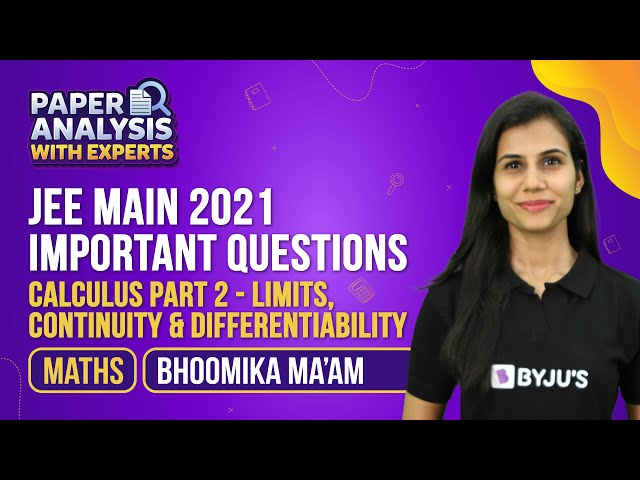### 12 Must-Do JEE Questions of Limits Continuity and Differentiability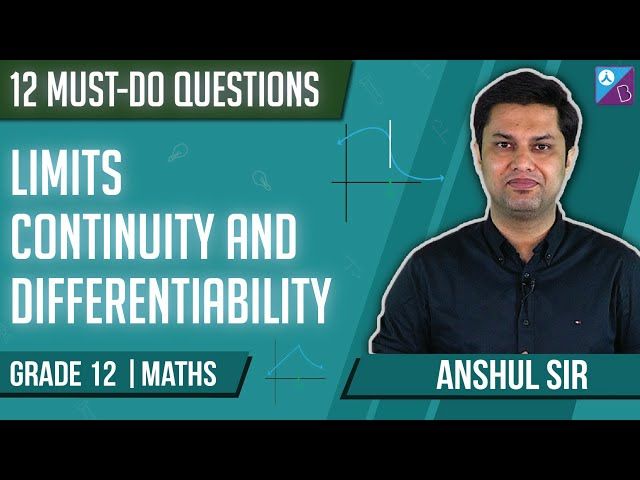## Differentiability

A function, say f(x), is said to be differentiable at the point x = a if the derivative f ‘(a) exists at every point in its domain.

### Existence of Derivative

Right and left-hand derivative

RHD: f’(0+) =

$$\begin{array}{l}\underset{h\to {{0}^{+}}}{\mathop{\lim }} \frac{f(a+h)-(a)}{h}\end{array}$$

LHD: F’

$$\begin{array}{l}F'({a}^{-}) = \underset{h\to {{0}^{-}}}{\mathop{\lim }} \frac{h(a-h)-f(a)}{-h}\end{array}$$

#### How can a function fail to be differentiable?

The function f(x) is said to be non-differentiable at x = a, if

(a) Both RHD & LHD exist but are not equal

(b) Either or both RHD & LHD are not finite

(c) Either or both RHD & LHD do not exist.

### Limits, Continuity and Differentiability – Part 1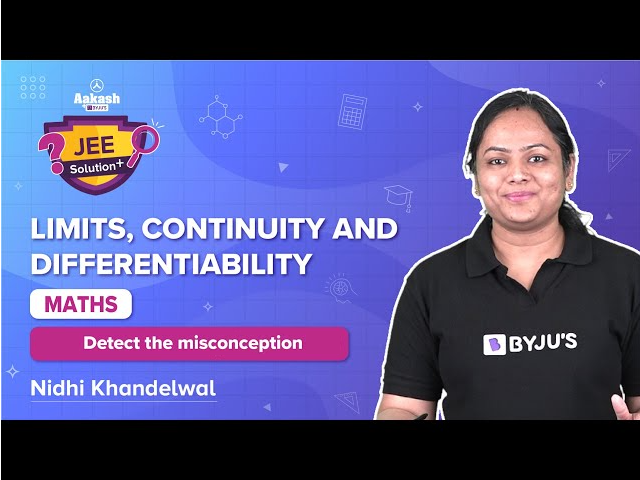### Limits, Continuity and Differentiability – Part 2### Limits, Continuity and Differentiability – Important Topics### Limits, Continuity and Differentiability – Important Questions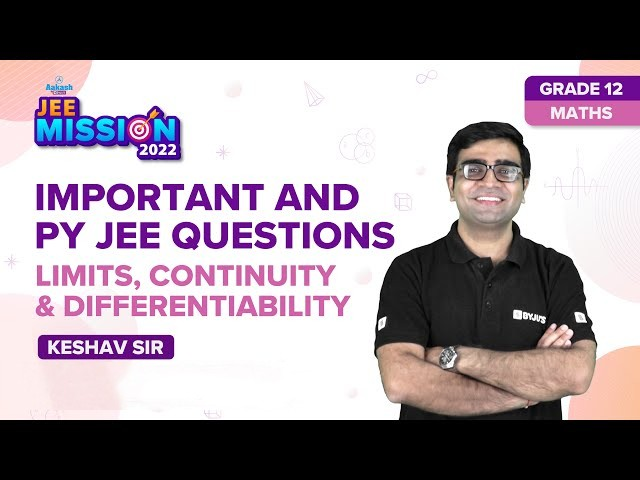### Limits, Continuity and Differentiability – Revision Lesson Part 1### Limits, Continuity and Differentiability – Revision Lesson Part 2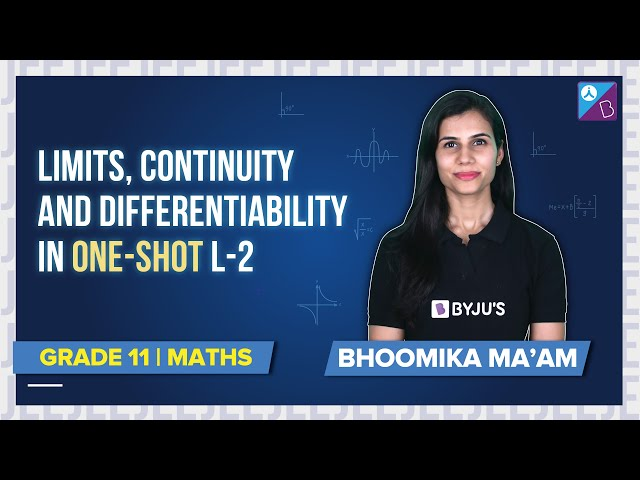### Continuity of a Function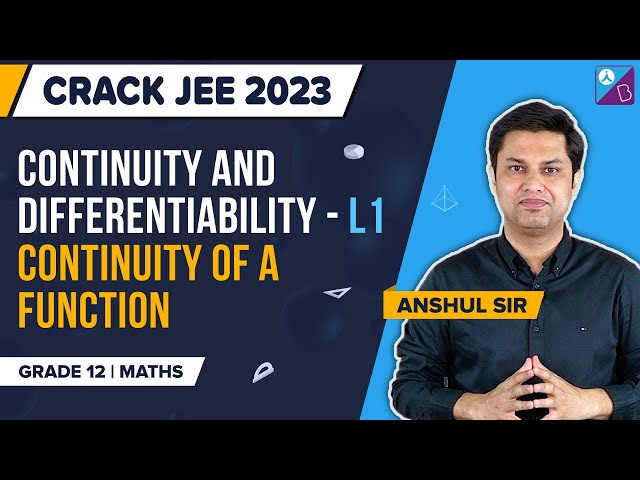### Theorems on Continuity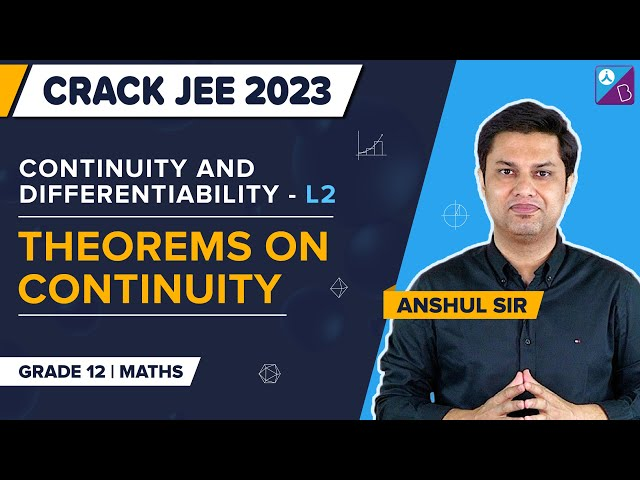### Differentiability and Its Conditions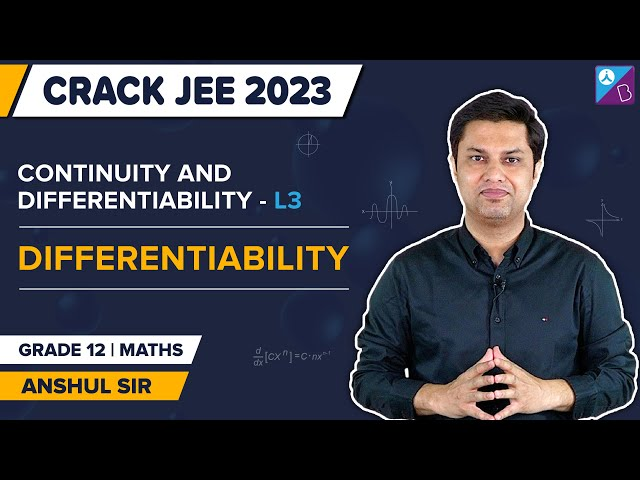### Differentiability in an Interval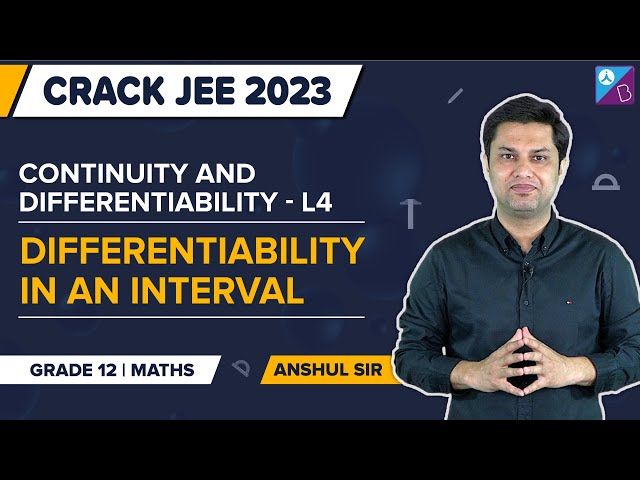Q1

### Give the definition of continuous function in Mathematics.

A function is said to be continuous at a point x = a, if limx→a f(x) exists and limx→a f(x) = f(a).

Q2

### What do you mean by differentiability?

A function f(x) is said to be differentiable at the point x = a if the derivative f’(a) exists at every point in its domain.

Q3

### How do you check the continuity of a function f(x) at x = a?

We have to check the left-hand limit and right-hand limit and the value of the function at a point x=a. If LHL = RHL = value of the function at x = a, then the function is continuous at x = a.

Q4

### What do you mean by the second derivative of a function?

The second derivative is the derivative of a function. We denote the second derivative of f(x) by f’’(x).

Q5

### Are continuity and differentiability related?

Yes, if a function is differentiable at any point in its domain, it will be continuous at that point; vice versa, it is not always true.

Q6

### What is the value of limx→ 0(log (1+x)/x)?

The value of limx→ 0(log (1+x)/x) = 1.

Q7

### What is the value of  limx→ 0 (ex-1)/x?

The value of limx→ 0 (ex-1)/x = 1.

Q8

### Is a continuous function always differentiable?

A continuous function need not be differentiable. If a function f(x) is differentiable at point a, then it is continuous at point a. But the converse is not true.